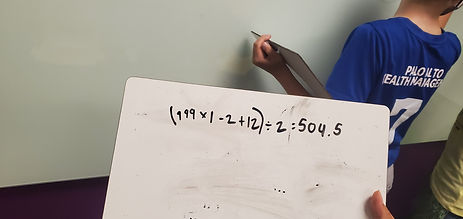Mr. Jake

Target 1​

Lesson Type:

New

Data Probability

:

Interpreting Data

Construct and read graphs from given information to solve problems.

1:

Construct and read scaled line graphs.

5th

Vocabulary:

Line Graph, Data, Axis, Scale

Activities:

Collected data and created line graphs with the information

Answer questions based on information found in a line graphHome Exploration

Guiding Questions:Absent Students:

Target 2

:

1:

Solve problems that have more than one operation.

2:

Define PEMDAS – Parenthesis, Exponents, Multiplication, Division, Addition, Subtraction.

3:

Understand that calculating in the wrong order can result in a wrong answer.

4:

Correctly identify the order of operations and use them to simplify expressions.

5:

Understand that the presence of parenthesis in an arithmetic equation signals for this part of the problem to be solved first.

6th

Vocabulary:

Operation, Arithmetic

Activities:

Practiced using the order of operations to solve problems

Created multi step problems for friends to solveHome Exploration

Guiding Questions:Target 3

:

Vocabulary:

Activities:Home Exploration